# Motion in One Dimension: JEE Main(Part- 3) - Physics, Solution by DC Pandey Notes | Study DC Pandey Solutions for JEE Physics - JEE

## JEE: Motion in One Dimension: JEE Main(Part- 3) - Physics, Solution by DC Pandey Notes | Study DC Pandey Solutions for JEE Physics - JEE

The document Motion in One Dimension: JEE Main(Part- 3) - Physics, Solution by DC Pandey Notes | Study DC Pandey Solutions for JEE Physics - JEE is a part of the JEE Course DC Pandey Solutions for JEE Physics.
All you need of JEE at this link: JEE

Graphs

Ques 28: Displacement-time graph of a particle moving in a straight line is as shown in figure.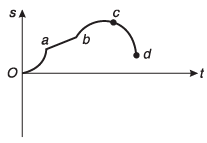(a) Find the sign of velocity in regions oa, ab, be and cd.
(b) Find the sign of acceleration in the above region.
Ans: (a) positive, positive, positive, negative (b) positive, zero, negative, negative
Sol: OA : slope is + ive and increasing.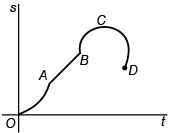∴ velocity is + ive and acceleration is + ive.
AB : slope is + ive and constant
∴ velocity is +ive and acceleration is zero.
BC : sope is + ive and decreases.
∴ velocity is + ive and increasing.
CD : slope is –ive and increasing
∴ velocity is - ive and acceleration is - ive.

Ques 29: Let us call a motion as:
M1 → if velocity and acceleration both are positive.
M2 → if velocity is positive but acceleration is negative.
M→ if velocity and acceleration both are negative.
M4 → if velocity is negative but acceleration is positive.

(a) State, in which of the above four motions, magnitude of velocity is increasing and in which it is decreasing.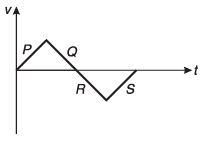(b) v-t graph of a particle moving in a straight line is as shown in figure. The whole graph is made up of four straight lines P, Q, R and S. These four straight lines indicate four type of motions (M1.. , M4) discussed above. State, which straight line corresponds to which type of motion.
Ans: (a) In M1 and M3 magnitude is increasing, in M2 and M4 magnitude is decreasing
(b) P → M1; Q → M2; R → M3; S → M4
Sol: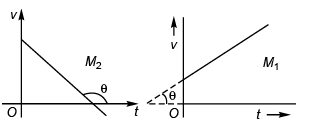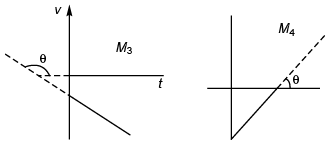In M1 and M3 : 0° < θ < 90°
∴ slope is + ive i.e., acceleration is + ive.
In M2 and M4 : 90° < θ < 180°
∴ slope is - ive i.e., acceleration is - ive.
(a) M1 : Magnitude of velocity is increasing.
M2 : Magnitude of velocity is decreasing.
M3 : Magnitude of velocity is increasing.
M4 : Magnitude of velocity is decreasing.
M1 and M3.
(b) P → M1
Q → M2
R → M3
S → M4

Ques 30: Velocity-time graph of a particle moving in a straight line is shown in figure. At time t = 0, s = - 10 m. Plot corresponding a-t and s-t graphs.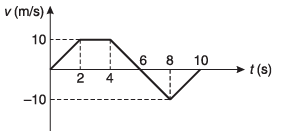Ans: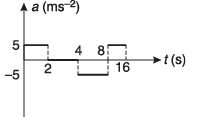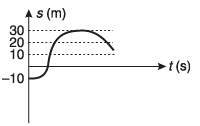Sol: Case I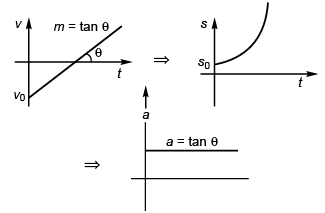v = mt - v0 (st-line)
∴ ∫ ds = ∫ (mt - v0) dt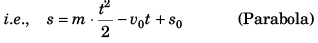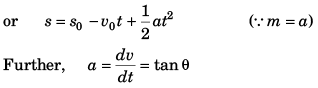As for 0° < q < 90°
tan θ is + ive, a is + ive.
Case II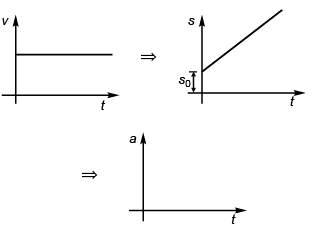v = k (constant) (st line parallel to time-axis)
⇒  ∫ ds = ∫ k dt
s = kt + s0
Further, a = dv/dt = 0
Case III.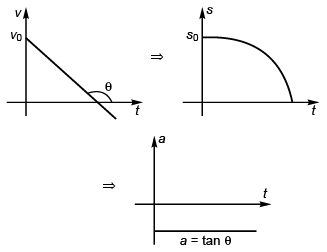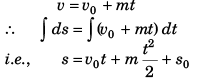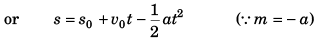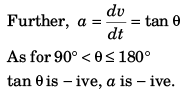Time-displacement graph Time Areaunder the graph InitialDisplacement Netdisplacement at 0 s 0 m - 10 m - 10 m 2 s 10 m - 10 m 0 m 4 s 30 m - 10 m + 20 m 6 s 40 m - 10 m + 30 m 8 s 30 m - 10 m + 20 m 10 s 20 m - 10 m + 10 m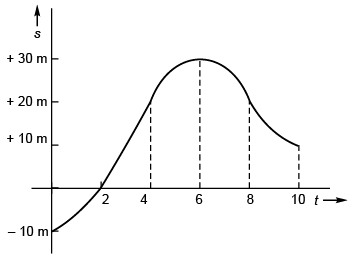Time-acceleration graph time slope acceleration 0 - 2 s 5 5 m/s2 2 - 4 s zero 0 m/s2 4 - 6 s - 5 - 5 m/s2 6 - 8 s - 5 - 5 m/s2 8 - 10 s + 5 + 5 m/s2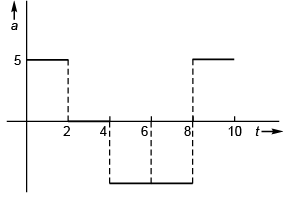Ques 31: Velocity-time graph of a particle moving in a straight line is shown in figure. At time t = 0, s = 20 m. Plot a-t and s-t graphs of the particle.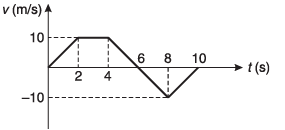Ans: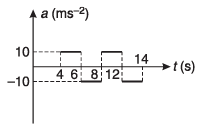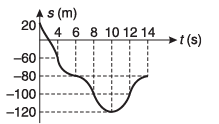Sol: a = slope of v-t graph.
S = area under v-t graph.
Corresponding graphs are drawn in the answer sheet.

Ques 32: Velocity-time graph of a particle moving in a straight line is shown in figure. In the time interval from t = 0 to t = 14s, find: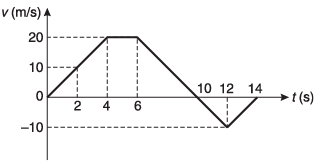(a) average velocity and
(b) average speed of the particle
Ans: (a) (50/7) ms-1
(b) 10 ms-1
Sol: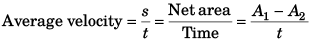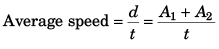Ques 33: Acceleration-time graph of a particle moving in a straight line is as shown in figure. At time t = 0, velocity of the particle is zero. Find: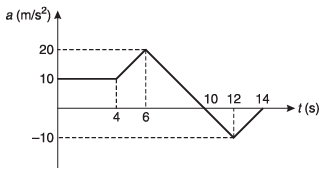(a) average acceleration in a time interval from t = 6s to t = 12s,
(b) velocity of the particle at t = 14s.
Ans: (a) 5 ms-2
(b) 90 ms-1
Sol: Average acceleration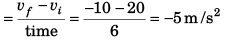vf - vi = Area under a-t graph.

Ques 34: Velocity-time graph of a particle moving in a straight line is shown in figure. At time t = 0, displacement of the particle from mean position is 10 m. Find: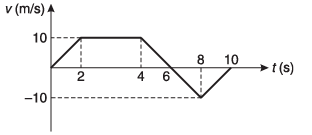(a) acceleration of particle at t = Is, 3s and 9 s.
(b) position of particle from mean position at t = 10s.
(c) write down s-t equation for time interval:
(i) 0 < / < 2s,
(ii) 4s < t < 8s
Ans: (a) 5 ms-2, zero, 5 ms-2
(b) s = 30 m
(c) (i) s = 10 + 2.5 t2
(ii) s = 40 + 10 (t - 4) - 2.5 (t - 4)2
Sol: (a) Acceleration = slope of v-t graph.
(b) rf - ri = s = area under v-t graph.
(c) Equations are written in answer sheet.

The document Motion in One Dimension: JEE Main(Part- 3) - Physics, Solution by DC Pandey Notes | Study DC Pandey Solutions for JEE Physics - JEE is a part of the JEE Course DC Pandey Solutions for JEE Physics.
All you need of JEE at this link: JEEUse Code STAYHOME200 and get INR 200 additional OFF

## DC Pandey Solutions for JEE Physics

210 docs

Track your progress, build streaks, highlight & save important lessons and more!

,

,

,

,

,

,

,

,

,

,

,

,

,

,

,

,

,

,

,

,

,

,

,

,

;